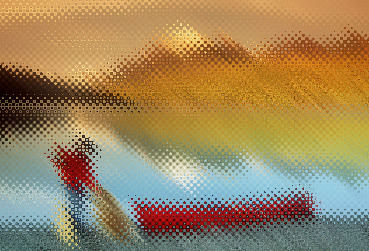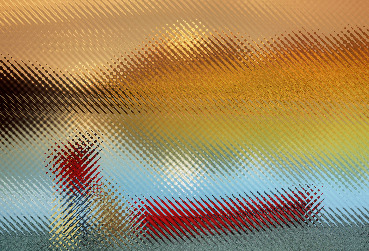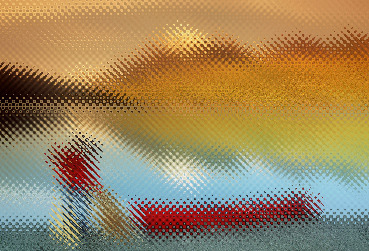## SCREENEFFECTS

 Applies screen-like effects to an image.

 USAGE: screeneffects [-s spacing] [-r] [-t type] [-a amount] [-m method] [-c color] infile outfile USAGE: screeneffects [-h or -help] -s ... spacing ... pixel spacing of screen pattern; float>0; default=6 -r ............... rotate screen by 45 degrees -t ... type ...... screen type; simple (or s) or displace (or d); .................. default=simple -a ... amount .... displacement amount; float>0; default=6 -m ... method .... displacement method; 1, 2 or 3; 1 indicates combine .................. x and y displacement maps; 2 indicates to use x and y .................. maps separately for the x and y displacements; 3 .................. indicates to use x and y maps for y and x displacements; .................. default=0 -c ... color ..... simple method screen colorization; default=black PURPOSE: To apply screen-like effects to an image. DESCRIPTION: SCREENEFFECTS applies screen-like effects to an image. The screen effects may be either simple or displacement. In the former, a screen pattern is mixed with the image and can be colored. In the latter, the screen pattern comes from various image displacement patterns. The screen pattern is generated from a sine wave pattern along x and another along y. Then they are optionally rotated 45 degrees. ARGUMENTS: -s spacing ... SPACING is the approximate pixel spacing of the screen pattern. Values are floats>0. The default=6 -r ... ROTATE screen by 45 degrees from x and y orientation. Nominally, one gets more appealing results with no rotation for type=simple and with rotation for type=displace. -t type ... TYPE is screen type. Choices are simple (or s) and displace or (d). With type=simple, the screen pattern is mixed with the image and the screen can be colored. With type=displace, the screen texture comes from a displacement of the image. The default=simple. -a amount ... AMOUNT is the pixel displacement amount for type=displace. Values are floats>0. The default=6 -m method ... METHOD is the displacement method. Choices are: 1, 2 or 3. Two displacement maps are created from sine waves along the x and along the y direction. Each may then be rotated 45 degrees. With method=1, the x and y displacement maps are merged and the merged map is used for the both the x and y displacements. With method=2, the x and y displacement maps are not merged and are used for the x and y displacements, respectively. With method=3, the displacement maps are swapped and then used for the x and y displacements, respectively. The default=1. -c color ... COLOR is the screen color when type=simple. Any valid IM color specification is allowed. The default=black. See http://imagemagick.org/script/color.php CAVEAT: No guarantee that this script will work on all platforms, nor that trapping of inconsistent parameters is complete and foolproof. Use At Your Own Risk.

### EXAMPLES

 Example 1 Original Arguments: -s 6 -t simple -c black Arguments: -s 6 -t simple -c blue Arguments: -s 3 -t displace -a 6 -r -m1OriginalArguments: -s 6 -t simple -c blackArguments: -s 3.5 -t displace -a 10 -r -m 1Arguments: -s 3.5 -t displace -a 10 -r -m 2Arguments: -s 3.5 -t displace -a 10 -r -m 3What the script does is as follows: Creates a sinusoidal image along x Creates a sinusoidal image along y Merges the sinusoidal images if desired Rotates the sinusoidal image(s) if desired by 45 degrees Uses the sinusodial image(s) to composite with the input or as a displacement map wth the input This is equivalent to the following IM commands ww=`convert \$infile -ping -format "%w" info:` hh=`convert \$infile -ping -format "%h" info:` maxdim=`convert xc: -format "%[fx:sqrt(2)*max(\$ww,\$hh)]" info:` xf=`convert xc: -format "%[fx:\$ww/\$spacing]" info:` yf=`convert xc: -format "%[fx:\$hh/\$spacing]" info:` avef=`convert xc: -format "%[fx:0.5*(\$xf+\$yf)]" info:` xf=\$avef yf=\$avef if [ "\$rotation" = "no" ]; then convert -size \${hh}x\${ww} gradient: -rotate 90 \ -function sinusoid "\$xf,0,.5,.5" \$tmp1 convert -size \${ww}x\${hh} gradient: -rotate 180 \ -function sinusoid "\$yf,0,.5,.5" \$tmp2 elif [ "\$rotation" = "yes" ]; then convert -size \${maxdim}x\${maxdim} gradient: \ -distort SRT -45 \ -gravity center -crop \${ww}x\${hh}+0+0 +repage \ -contrast-stretch 0 -function sinusoid "\$xf,0,.5,.5" \$tmp1 convert -size \${maxdim}x\${maxdim} gradient: \ -distort SRT 45 \ -gravity center -crop \${ww}x\${hh}+0+0 +repage \ -contrast-stretch 0 -function sinusoid "\$yf,0,.5,.5" \$tmp2 fi if [ "\$type" = "simple" ]; then convert \$tmp1 \$tmp2 -average -contrast-stretch 0 \$tmp1 convert \$infile \( \$tmp1 +level-colors \${bcolor},white \) \$tmp1 \ -compose multiply -composite \$outfile elif [ "\$type" = "displace" -a "\$method" = "1" ]; then convert \$tmp1 \$tmp2 -average -contrast-stretch 0 \$tmp1 convert \$tmp1 \${inname}_mask.png composite -displace \${amount}x\${amount} \$tmp1 \$infile \$outfile elif [ "\$type" = "displace" -a "\$method" = "2" ]; then composite -displace \${amount}x\${amount} \$tmp1 \$infile \$tmp2 \$outfile elif [ "\$type" = "displace" -a "\$method" = "3" ]; then convert \$tmp1 \$tmp2 -average -contrast-stretch 0 \$tmp1 composite -displace \${amount}x\${amount} \$tmp2 \$infile \$tmp1 \$outfile fi